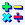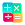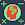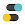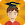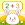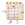# Math games: arithmetic, times tables, mental math

by Standy Software
1,000,000+ installs

Android application Math games: arithmetic, times tables, mental math developed by is listed under category Game Educational. According to Google Play Math games: arithmetic, times tables, mental math achieved more than 1,000,000 installs. Math games: arithmetic, times tables, mental math currently has 6,758 ratings with average rating value of 4.3. The current percentage of ratings achieved in last 30 days is 3.0%, percentage of ratings achieved in last 60 days is 6.13%. Math games: arithmetic, times tables, mental math has the current market position #41316 by number of ratings. A sample of the market history data for Math games: arithmetic, times tables, mental math can be found below. Last update on 2020-02-10.

Title: Math games: arithmetic, times tables, mental math Standy Software Game Educational Free Android
Total ratings: 6758 3.0% 6.13% 4.3
Installs (achieved): 1,000,000+ 1,298,788
5 star ratings: 4,639 897 443 181 595

### Screenshots

2019-02-17: Android application Math games: arithmetic, times tables, mental math achieved 1,000,000 installs. Android application Math games: arithmetic, times tables, mental math achieved 500,000 installs. Android application Math games: arithmetic, times tables, mental math achieved 100,000 installs. Android application Math games: arithmetic, times tables, mental math achieved 50,000 installs. Android application Math games: arithmetic, times tables, mental math achieved 10,000 installs. Android application Math games: arithmetic, times tables, mental math achieved 5,000 installs.

Total number of ratings
Total number of active users rated for Math games: arithmetic, times tables, mental math.

Total number of installs (*estimated)
Estimation of total number of installs on Google Play. Approximated from number of ratings and install bounds achieved on Google Play.

Average rating
Average rating value on Google Play. Given by active users of the application.

Ratings count comparing to the market leading app.

Rating distribution
Rating distribution given by active users.

Price history stats
History statistics on app prices for Math games: arithmetic, times tables, mental math.

Related apps